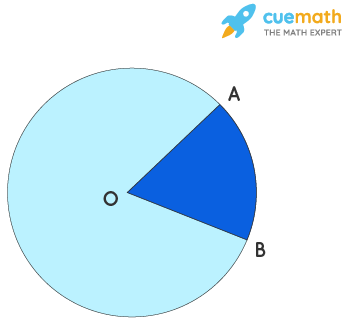# Central Angle Calculator

## What is Central Angle Calculator?

'Cuemath's Central Angle Calculator' is an online tool that helps to calculate the central angle for a given arc length and radius. Cuemath's online Central Angle calculator helps you to calculate the central angle in a few seconds.

## How to Use Central Angle Calculator?

• Step 1: Enter the arc length and radius in the given input box.
• Step 2: Click on the "Calculate" button to find the central angle for a given arc length and radius.
• Step 3: Click on the "Reset" button to find the central angle for different values.

## How to Find Central Angle?

A central angle is defined as the angle subtended by an arc at the center of a circle. The radius vectors form the arms of the angle. A central angle is calculated using the formula:

Central Angle = Arc length(AB) / Radius(OA) = (s × 360°) / 2πr, where 's' is arc length, and 'r' is radius of the circle.### Solved Example:

Find Central Angle if the arc length is 5 units and radius is 6 units?

### Solution:

Central Angle = Arc length(AB) / Radius(OA) = (s × 360°) / 2πr

= (5 × 360°) / 2 × 3.14 × 6

= 1800 / 37.68

= 47.77

Similarly, you can try the calculator to find the central angle for

1) arc length = 11units and radius = 9units

2) arc length = 7units and radius = 8units